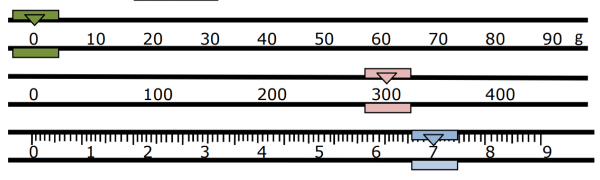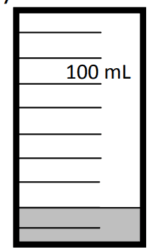# Math Worksheets Land

Math Worksheets For All Ages

# Math Worksheets Land

Math Worksheets For All Ages

# Liquid Volume and Mass Word Problems Worksheets

As you begin to work on more science-based activities you will come across these two measures. Science tells us that when a substance is found in different phases (solid, liquid, gas) the particles of matter become more spread out. As a result, we consider dry (solid) and liquid volumes to be different measures and they are recorded and measured differently. In this section we will demonstrate how to determine both of these measures with common laboratory equipment. We will also explore these measures in word problem form and show you strategies for solving these types of problems. These worksheets will have students learn how to determine the answer to word problems that include liquid volume and mass.

### Aligned Standard: Grade 3 Measurement - 3.MD.2

• Answer Keys - These are for all the unlocked materials above.

### Homework Sheets

A sheet on each skill liquid volume and mass problems. We also estimate mass, volume, and capacity.

• Homework 1- Karen, Jordan, and Kelly were collecting juice boxes. Each juice box holds 8 oz. Karen had 2, Jordan had 3, and Kelly had 4 juice boxes. How many ounces of juice did they have all together?
• Homework 2- Jeff and Gilbert get on the scale and weigh 175 pounds together. After Jeff gets off, Gilbert weighs 92 pounds. How much does Jeff weigh?
• Homework 3- Circle the best estimate for the mass of each object.

### Practice Worksheets

The sheets all start off simple and get harder in the middle. They ease up towards the end.

• Practice 1- You have a 500 mL container of lemonade. You pour a large glass of lemonade out of the container. The container now has 350 mL of lemonade. How much lemonade is in your glass?
• Practice 2- Silver bars weigh 7 pounds each. They are stacked 12 bars at a time. What is the weight of a silver stack?

### Math Skill Quizzes

I cannot tell you how many times answer kilometers for the length of the car...

• Quiz 1- Jeff has a 28-pound bag filled with large ketchup bottles. Each bottle weighs 4 pounds. How many bottles of ketchup does Jeff have?
• Quiz 2- A pail is filled with water. Is the pail more likely to hold 1,000 gallons or 1,000 mL of water?

### What is Liquid Volume and Mass?

Mass and its Units

Mass is the amount of matter that a body contains. All bodies have mass. The greater the amount of matter, the greater the amount of mass. Rocks, balls, cars, air, mountains, water, and everything that makes up the planet and the universe has mass.

The amount of mass of a solid, liquid, or gaseous object is determined with the scales. On Earth the mass and weight of objects are equal. A balance makes it possible to compare the known weight of a body against that of an unknown weight. The type of balance used depends on the type of object or material whose weight you want to measure.

The unit for measuring mass established by the International System of Units (SI), an organization that determines standard measures and units, is the kilogram (kg). Other units of mass are the gram (g), which is equivalent to 1/1000 kg; that is, one kilogram divided by 1000 equal parts, and the ton (t), which is equal to 1000 kg.

Measuring Mass with a Triple Beam Balances

One of the most common tools for accurately measuring mass under 500 grams is a triple beam balance. Because of this tool has a low degree of error you with find it used in many different science and engineering based activities. As the name indicates there are three beams that must be read it order to determine the mass of an object. You simply place the object you wish to weigh on the pan or cup that is present. You then push the middle slider (100 g interval) until it dips below the balance, then rest back one interval. You continue this same procedure with the top slider (10g interval). When you have those two-slider measures, it all comes down to the bottom slider that you move slowly until it balances in the center.The fun comes when you actually have to read the mass on the triple beam balance. This is actually just expanded form math. You have three beams that you must simply add to determine the full mass. If we look at the mass reading above. We will read this from the top-down: 10 g interval = 0 g, 100 g interval = 300 g, 1 g interval = 7 g. From here we would simply add the value on these to beams to determine the total: 0g + 300g + 7g = 307g. The balance above read 307 grams.

Volume and its Units

Matter occupies a place in space, which is measured in three dimensions. This three-dimensional space occupied by a quantity of matter is known as volume. A simple grain of sand has volume, the same as an apple, a brick, a person, a mountain, and a planet. Also, the air and any gas occupy volume. When you breathe, air is inhaled and as the lungs fill, you feel and see how the volume of the chest increases.

The unit of the International System of units for measuring volume is the cubic meter (m3). A cubic meter is the space occupied by a box one meter long, one meter wide, one meter high (1m x 1m x 1m). To measure smaller volumes, it is convenient to use the cubic centimeter (cm3) which is 1/1000000 m3.

The Concept of Liquid Volume

The volumetric containers are graduated to measure volumes in the chemical laboratory; they can be made of glass or plastic and have a scale engraved, almost always in milliliters (ml) or cubic centimeters (cm3). Both units correspond to one thousandth of a liter. In some volumetric measuring instruments, cm3 is expressed as cc.

Measuring Liquid Volume with a Graduated Cylinder

Graduated cylinders are one of the most common pieces of laboratory equipment that you will find. They are great for determining liquid volume of most substances. You just pour the liquid into the graduated cylinder to begin reading the volume. The first thing you must do before reading volume is determine the scale that cylinder is set upon. If we were going to read the volume of the cylinder below, you can see that the total volume of the cylinder is listed as 100 milliliters. Since there are 10 marks on the entire cylinder, this means that each mark indicates a 10 mL (100/10) volume. If we were asked to read the volume present in this cylinder it covers 2 marks, so it would be a 20 mL volume of liquid.Unlock all the answers, worksheets, homework, tests and more!
Save Tons of Time! Make My Life Easier Now

## Thanks and Don't Forget To Tell Your Friends!

I would appreciate everyone letting me know if you find any errors. I'm getting a little older these days and my eyes are going. Please contact me, to let me know. I'll fix it ASAP.